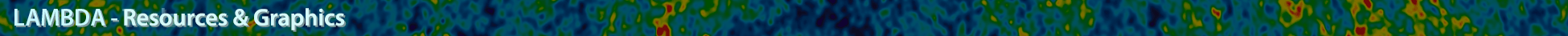# CMB B-mode Plot Data Sources

Here is a text file containing the data points used to generate the plot. The data are for l(l+1)Cl/2π, and are supplied in μK2.

ACTPol

• The Atacama Cosmology Telescope: A Measurement of the Cosmic Microwave Background Power Spectra at 98 and 150 GHz Choi, S. K. et.al. 2020, arXiv:2007.07289. ADS
The plotted limit for each bin was calculated from the part of the Gaussian likelihood function corresponding to C_l > 0.

BICEP1

BICEP2

BICEP2+Keck

• BICEP2/Keck Array X: Constraints on Primordial Gravitational Waves using Planck, WMAP, and New BICEP2/Keck Observations through the 2015 Season, Keck Array, BICEP2 Collaborations 2018, astro-ph

• Plotted points are the CMB component from a decomposition of the BB spectrum into CMB, dust, and synchrotron components. For the first bin, the CMB BB value is zero so the 95% confidence upper limit is plotted.

BICEP2+Keck/Planck

• A Joint Analysis of BICEP2/Keck Array and Planck Data BICEP2/Keck, et.al., astro-ph

• Plotted points are the dust-subtracted B-mode power as shown in figure 12 of the joint analysis paper and the plotted uncertainties are from the lensed-LambdaCDM+noise+dust simulations. The uncertainty values were provided by C. Pryke. For the first bin where the dust-subtracted BB is negative, we plot a 95% confidence upper limit calculated from the part of the Gaussian likelihood function corresponding to C_l > 0.

BOOMERANG

• A Measurement of the CMB EE Spectrum from the 2003 Flight of BOOMERANG T.E. Montroy, et al., 2006, ApJ, 647, 813M

• Plotted limits are max(0,BB) + 2*sigma_BB. BB and sigma_BB from NA pipeline results in Table 1 of Montroy et al., also in
B03_NA_21July05.tar

CAPMAP

• New Measurements of Fine-Scale CMB Polarization Power Spectra from CAPMAP at Both 40 and 90 GHz CAPMAP Collaboration, et.al., 2008, ApJ, 684, 771B

• BB limits from Figure 18 of this paper were provided by C. Bischoff, CAPMAP_95pc_BB_limits.dat.

CBI

• Implications of the Cosmic Background Imager Polarization Data J.L. Sievers, et al., 2007, ApJ, 660, 976S

• CBIpol_2.0_final.newdat
Plotted limits are max(0,BB) + 2*sigma_BB

DASI

• DASI Three-Year Cosmic Microwave Background Polarization Results E.M. Leitch., et al., 2005, ApJ, 624, 10L

• leitch_table1.txt

MAXIPOL

• Wu et al. 2007, ApJ, 665, 55

• lmin, lmax, 95% upper limit: 151, 693, 90.25 uK^2

POLARBEAR

• A Measurement of the Cosmic Microwave Background B-Mode Polarization Power Spectrum at Sub-Degree Scales from 2 years of POLARBEAR Data,
The POLARBEAR Collaboration, astro-ph

• The plotted data are POLARBEAR bandpowers from Pipeline A given in their Table 4 and the plotted error bars show statistical uncertainties only.

• Improved measurements of the temperature and polarization of the CMB from QUaD The QUaD collaboration, et.al., 2009, ApJ, 705, 978B

QUIET-Q

QUIET-W

SPTpol

• Measurements of B-Mode Polarization of the Cosmic Microwave Background from 500 Square Degrees of SPTpol Data,
Sayre et al. 2019, astro-ph

WMAP

lowest l bin:

• Seven-Year Wilkinson Microwave Anisotropy Probe (WMAP) Observations: Power Spectra and WMAP-Derived Parameters
Larson, D., et.al., 2011, ApJS, 192, 16L
reprint (0.7 MB) / preprint (569 KB) / bundled figures (123 KB) / individual figures / ADS / astro-ph

• lmin, lmax, 95% upper limit: 2, 7, 0.055 uK^2

higher l bins:

A service of the HEASARC and of the Astrophysics Science Division at NASA/GSFCHEASARC Director: Dr. Andrew F. Ptak
LAMBDA Director: Dr. Thomas M. Essinger-Hileman
NASA Official: Dr. Thomas M. Essinger-Hileman
Web Curator: Mr. Michael R. Greason